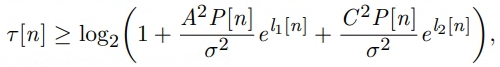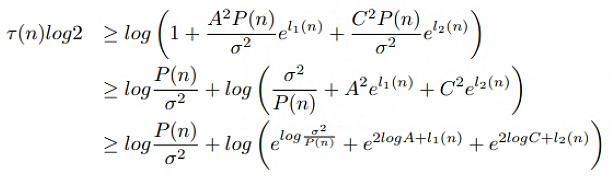Hello, everyone! Thank you for your attention !
Variable : tao[n] , l1[n] and l2[n]help log_sum_exp

log_sum_exp log(sum(exp(x))).
log_sum_exp(X) = LOG(SUM(EXP(X)).

``````When used in a CVX model, log_sum_exp(X) causes CVX's successive
approximation method to be invoked, producing results exact to within
the tolerance of the solver. This is in contrast to LOGSUMEXP_SDP,
which uses a single SDP-representable global approximation.

If X is a matrix, LOGSUMEXP_SDP(X) will perform its computations
along each column of X. If X is an N-D array, LOGSUMEXP_SDP(X)
will perform its computations along the first dimension of size
other than 1. LOGSUMEXP_SDP(X,DIM) will perform its computations
along dimension DIM.

Disciplined convex programming information:
log_sum_exp(X) is convex and nondecreasing in X; therefore, X
must be convex (or affine).
``````

You will need to divide by `log(2)` because `log_sum_exp` is based on `log`, not `log2`.

Despite what the help says, CVX’s Successive Approximation method is not used if CVX 2.2+Mosek9.x or 10.x are used (preferred), or if CVXQUAD’s `exponential.m` replacement is used (2nd choice to use of CVX 2.2 + Mosek 9.x or 10.x)I think you got it right, except in the first element of `X`, you forgot to square `sigma`, or multiply the log by 2, And of course the `ln` in that element should be `log`.